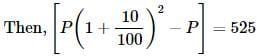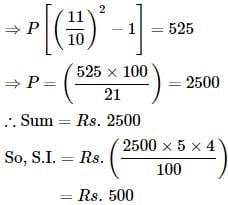Test: Compound Interest- 2

# Test: Compound Interest- 2

Test Description

## 10 Questions MCQ Test CSAT Preparation for UPSC CSE | Test: Compound Interest- 2

Test: Compound Interest- 2 for Banking Exams 2023 is part of CSAT Preparation for UPSC CSE preparation. The Test: Compound Interest- 2 questions and answers have been prepared according to the Banking Exams exam syllabus.The Test: Compound Interest- 2 MCQs are made for Banking Exams 2023 Exam. Find important definitions, questions, notes, meanings, examples, exercises, MCQs and online tests for Test: Compound Interest- 2 below.
Solutions of Test: Compound Interest- 2 questions in English are available as part of our CSAT Preparation for UPSC CSE for Banking Exams & Test: Compound Interest- 2 solutions in Hindi for CSAT Preparation for UPSC CSE course. Download more important topics, notes, lectures and mock test series for Banking Exams Exam by signing up for free. Attempt Test: Compound Interest- 2 | 10 questions in 10 minutes | Mock test for Banking Exams preparation | Free important questions MCQ to study CSAT Preparation for UPSC CSE for Banking Exams Exam | Download free PDF with solutions
 1 Crore+ students have signed up on EduRev. Have you?
Test: Compound Interest- 2 - Question 1

### The compound interest on a certain sum for 2 years at 10% per annum is Rs. 525. The simple interest on the same sum for double the time at half the rate percent per annum is:

Detailed Solution for Test: Compound Interest- 2 - Question 1

Let the sum be Rs. PTest: Compound Interest- 2 - Question 2

### The effective annual rate of interest corresponding to a nominal rate of 6% per annum payable half-yearly is:

Test: Compound Interest- 2 - Question 3

### The Simple interest on a certain sum for 2 years at 20% per annum is Rs. 80. The corresponding compound interest is

Detailed Solution for Test: Compound Interest- 2 - Question 3

P = (100×80)/(20×2) = 200
20%(200)= 40
20%(40) = 8
Required compound interest = 2(40)+ 8
= 88

Test: Compound Interest- 2 - Question 4

A sum put out at 4% compound interest payable half-yearly amounts to Rs. 13265.10 in 1 1/2 years. The sum is:

Test: Compound Interest- 2 - Question 5

Arun invested an amount of Rs. 20000 in a fixed deposit scheme for 2 years at compound interest rate 4 p.c.p.a. How much amount will Arun get on maturity of the fixed deposit?

Test: Compound Interest- 2 - Question 6

The compound interest on Rs. 20,480 at 6 1/4 % per annum for 2 years 73 days, is:

Test: Compound Interest- 2 - Question 7

A sum amounts to Rs. 882 in 2 years at 5% compound interest. The sum is

Detailed Solution for Test: Compound Interest- 2 - Question 7

Compound interest = Amount -Principal
Amount = P(1 + R/100)N
882 = P(1+5/100)2
=  882 × 20/21 × 20/21
P = 800

Test: Compound Interest- 2 - Question 8

Simple interest on a certain sum of money for 4 years at 5% per annum is half the compound interest on Rs. 3000 for 2 years at 10% per annum. The sum placed on simple interest is:

Detailed Solution for Test: Compound Interest- 2 - Question 8

C.I = P[(1 + r/100)n - 1]
C.I = 3000[(1 + 0.10)2 - 1]
= 3000(1.21 - 1)
⇒ 3000 * 0.21
⇒ 630
S.I = 630/2
⇒ 315
As we know that, P = (SI * 100)/(R * T)
⇒ (315 * 100)/(4 * 5)
⇒ 1575

Test: Compound Interest- 2 - Question 9

The least number of complete years in which a sum of money put out at 20% compound interest will be more than doubled is

Detailed Solution for Test: Compound Interest- 2 - Question 9

P [1 + (r/100)]n  >  2P
⇒ P [1 + (20/100)]n  >  2P
[1 + (2/10) ]n  > 2
[12 / 10]n  > 2
[6/5]n  > 2
{6/5 * 6/5 * 6/5 * 6/5}  >  2
∴ n = 4

Test: Compound Interest- 2 - Question 10

The difference between simple interest and compound on Rs. 2400 for one year at 10% per annum reckoned half-yearly is:

## CSAT Preparation for UPSC CSE

72 videos|64 docs|92 tests
 Use Code STAYHOME200 and get INR 200 additional OFF Use Coupon Code
Information about Test: Compound Interest- 2 Page
In this test you can find the Exam questions for Test: Compound Interest- 2 solved & explained in the simplest way possible. Besides giving Questions and answers for Test: Compound Interest- 2, EduRev gives you an ample number of Online tests for practice

## CSAT Preparation for UPSC CSE

72 videos|64 docs|92 tests